The options of fclust

2020-11-30

The function fclust can work using different methods and models managed by options. The three main options are opt.method, opt.model and opt.mean. Additional option is opt.jack. The options are defined by default for focusing on the main results provided by a functional clustering. They are: opt.tree = list(“prd”, “leg”), opt.perf = list(“prd”, “pub”), opt.motif = list(“obs”, “hor”, “leg”).

The option opt.method

opt.method determines the method of clustering. The option can be “divisive”, “agglomerative” or “apriori”. All methods generate hierarchical trees. Each tree is complete, running from a unique trunk to as many leaves as components.

• If opt.method = “divisive”, the components are clustered from the trivial cluster where all components are together, towards the clustering where each component is isolated in a cluster. This method is very efficient because the first component partioning change considerably the coefficient of determination of clustering model. The first divisions are thus very discriminative, and it is therefore possible to surely observe the effect on performance of co-occurring components within assemblages.

• If opt.method = “agglomerative”, the components are clustered from the trivial clustering where each component is isolated in a cluster, towards the cluster where all components are together. In most cases, the first component clustering do not change the coefficient of determination of clustering model: the first clustering are not discriminative and it is therefore not possible to select the component combinations associated with the strongest effects of assemblage performance.

res <- fclust(dat.2004, nbElt, opt.method = "divisive")
fclust_plot(res, opt.tree = list("prd"))

res <- fclust(dat.2004, nbElt, opt.method = "agglomerative")
fclust_plot(res, opt.tree = list("prd"))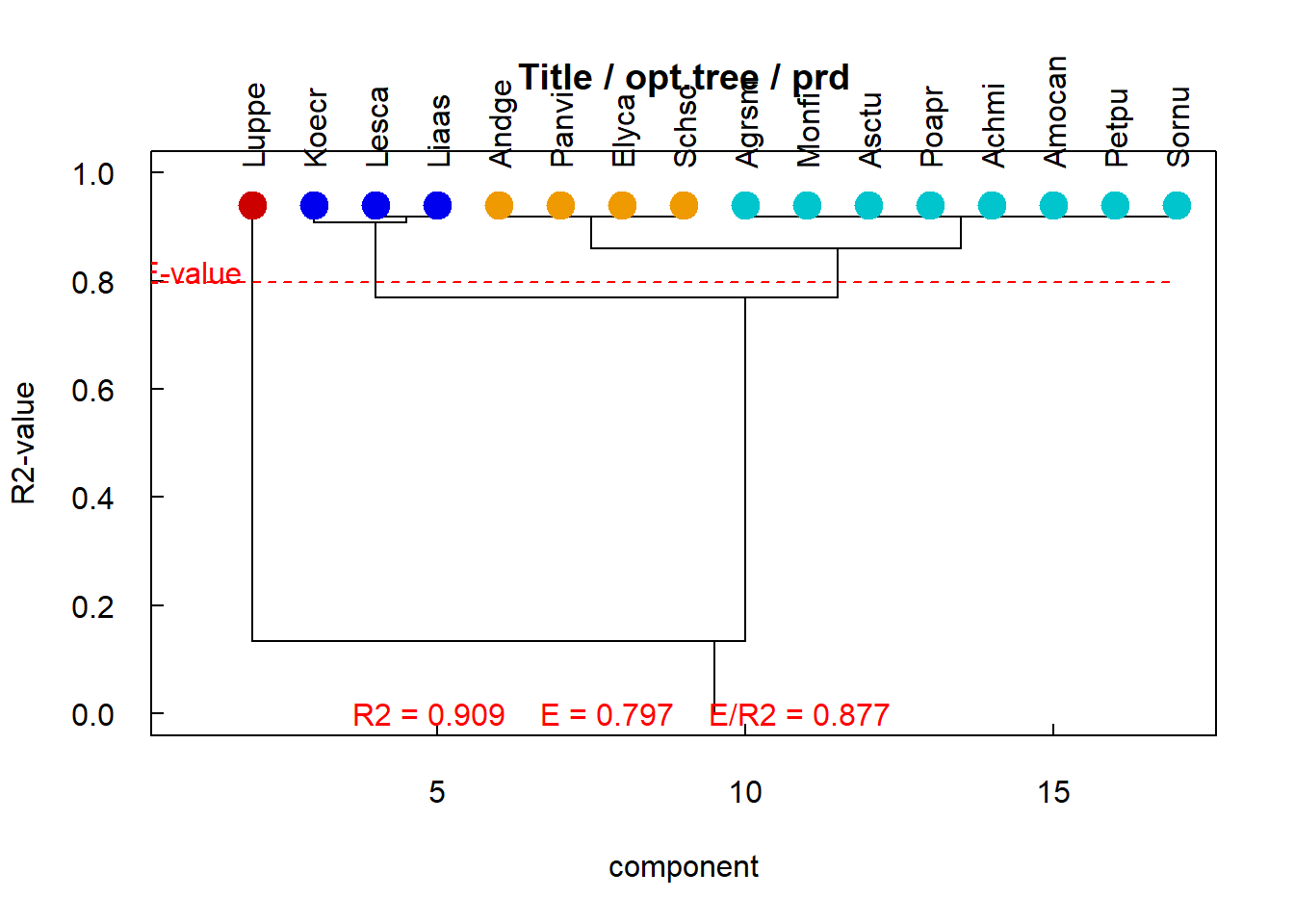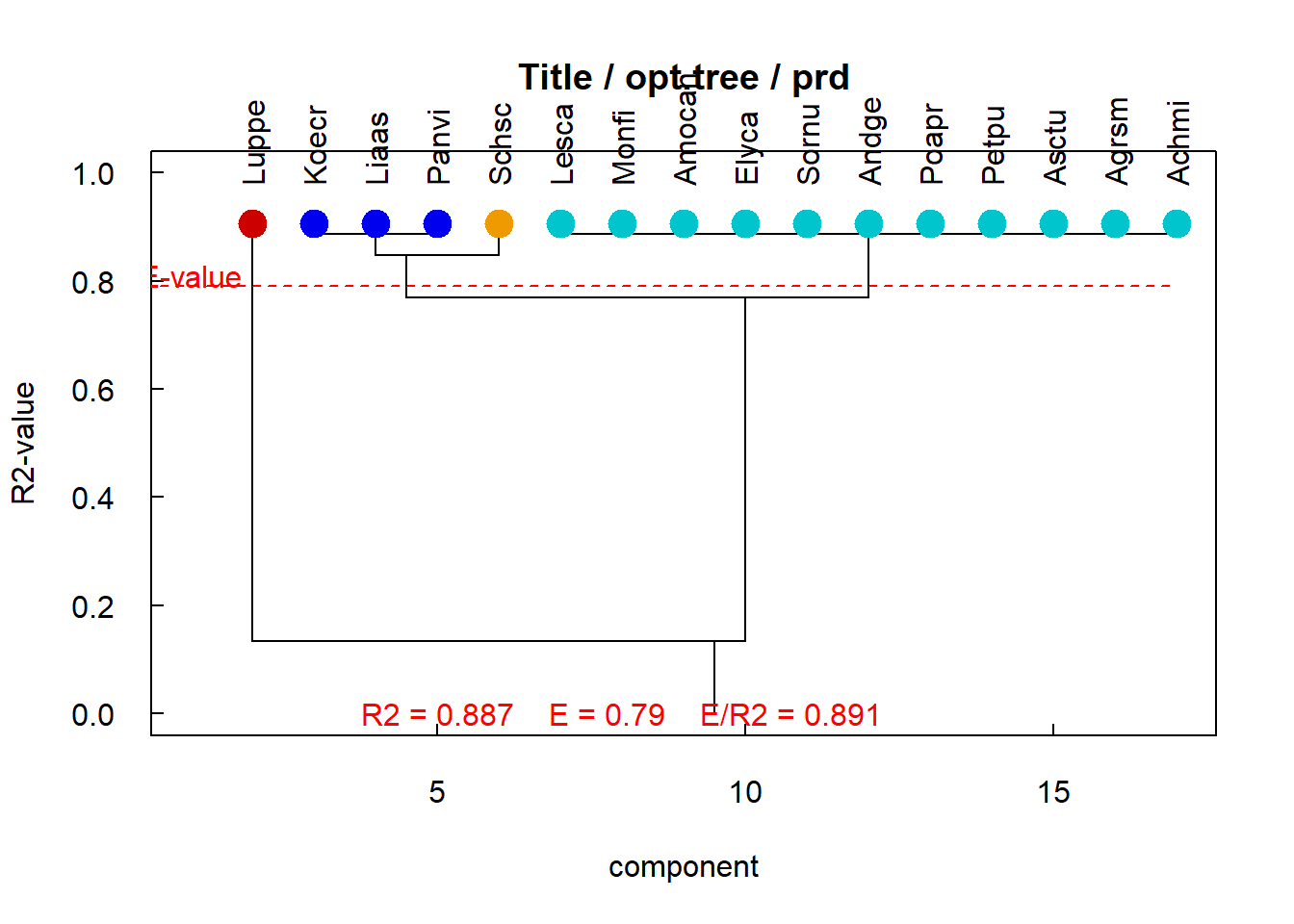Opt.method determines the method of clustering: on left ‘divisive’, on right ‘agglomerative’

The left graph shows the tree obtained using “divisive”" method, the right graph the tree obtained using “agglomerative”" method. Divisive method gives generally a more accurate and a more predictive tree than agglomerative method. Divisive and agglomerative methods give the same results whether the number of components is small, thus the number of possible component partitions also is small. (The possible component partitions is given by the number of Stirling of second species, see the function stirling).

• opt.method = “apriori” : the option assumes that the user knows an a priori partitioning of the system components he is studying. The partition is arbitrary, in any number of clusters of components, as long as it is complete, i.e. it includes as many components as the system includes. In this option, an a priori component partition, noted affectElt, should be provided. affectElt is a vector of integers or characters of length nbElt. A hierarchical tree is then built, by using opt.method = “divisive” from the a priori defined component partition towards the uppermost part of tree (towards tree leaves), and by using opt.method = “agglomerative” from the a priori defined component partition towards the lowest part of tree (towards tree trunk). The resulting tree is therefore forced by the given, a priori component partition affectElt.
Note that opt.method = “apriori” with affectElt = rep(1, nbElt) is equivalent to opt.method = “divisive”, since the a priori partitioning consists of a single cluster that brings together all the components of the system. In contrary, opt.method = “apriori” with affectElt = seq_len(nbElt) is equivalent to opt.method = “agglomerative”, since the a priori partitioning consists of isolating each component in a cluster.
apriori <- c(1,3,2,4,1,3,3,2,1,2,1,4,2,3,4,4)
apriori <- c("F","C3","L","C4","F","C3","C3","L","F","L","F","C4","L","C3","C4","C4")

res <- fclust(dat.2004, nbElt, opt.method = "apriori", affectElt = apriori)
fclust_plot(res, opt.tree = list("prd", "leg", cols = apriori))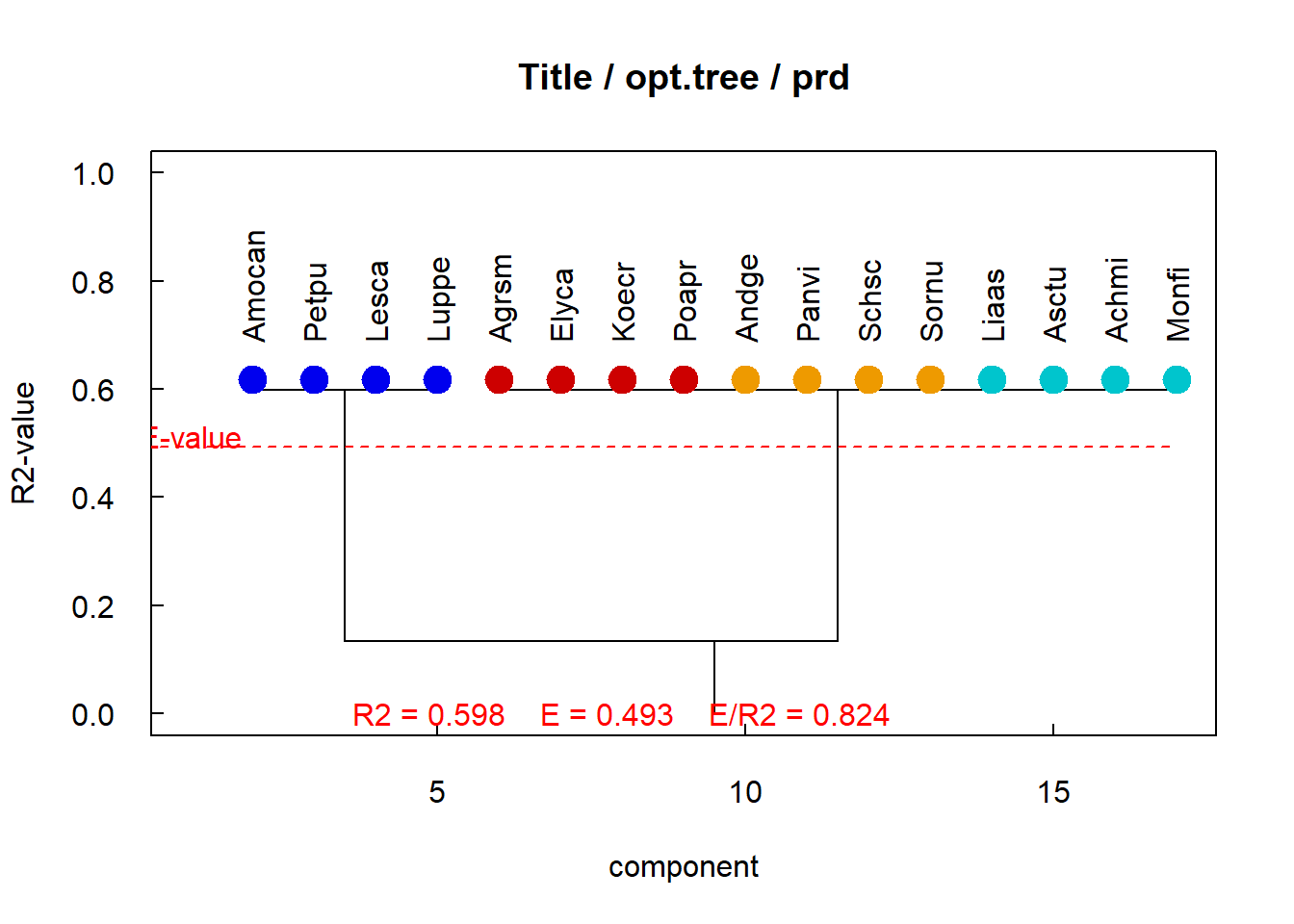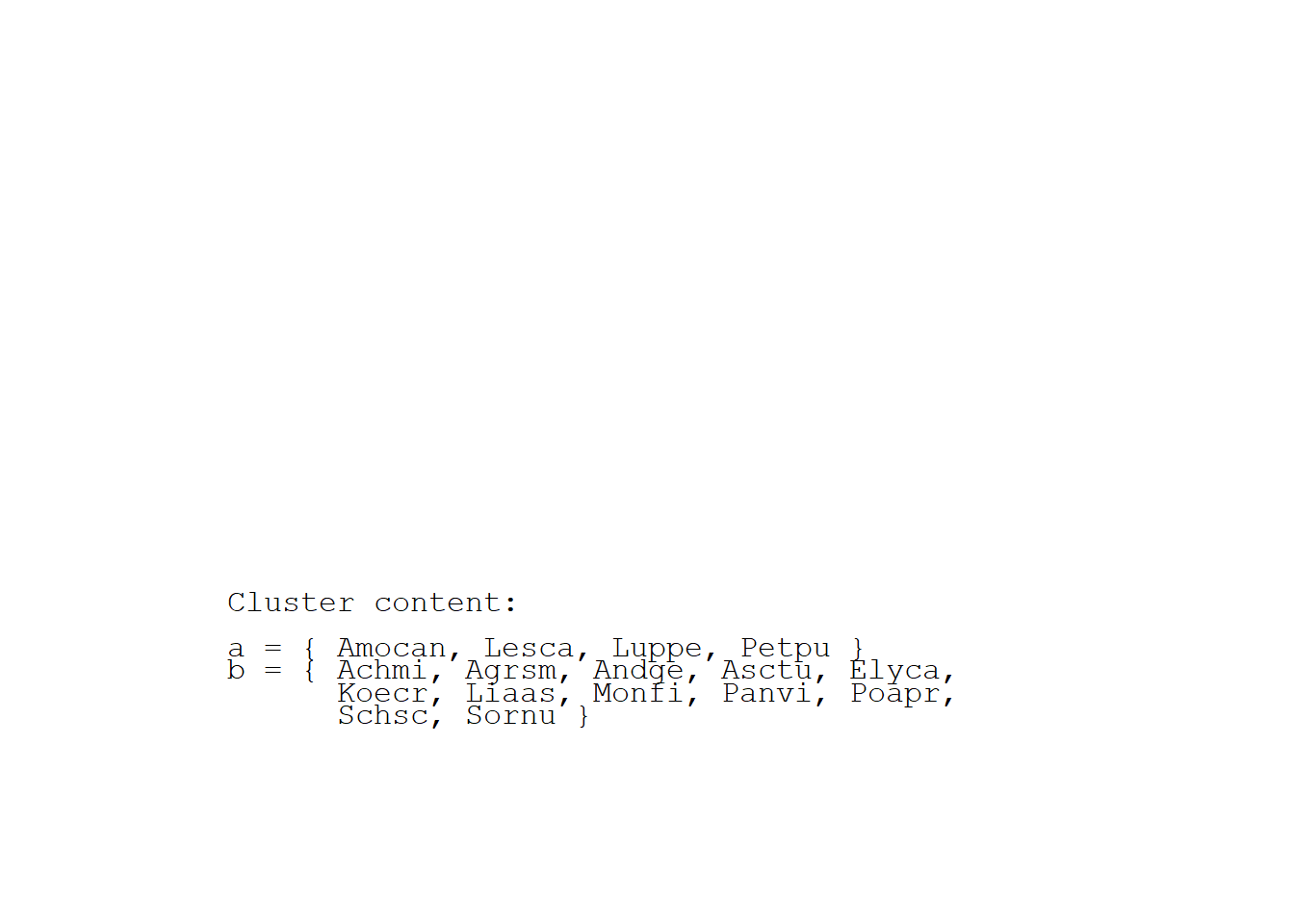Opt.method=‘apriori’ forces the hierarchical tree to include a given component partition

In ecology, meadow species are classically a priori clustered in Legumes (in red), Forbs (in cyan), C3-grasses (in blue) and C4 grasses (in gold). A functional clustering suggests that only the legumes group is pertinent.

The option opt.model

opt.model determines the model for predicting assemblage performance. The option can be “bymot” or “byelt”.

• opt.mod = “bymot”: the option is the simplest one. The performances are modelled as the mean performance of assemblages that share a same assembly motif, by including all assemblages that belong to the same assembly motif. Consequently, all assemblages that share a same assembly motif have the same predicted performance, whatever their component composition. The modelling of assemblage performances is therefore based only on the partitioning of components into functional groups, and the resulting partitioning of assemblages into assembly motifs. It does not take into account the fact that the elementary composition of each assemblage differs from one assemblage to another within the same assembly motif, and that these elementary compositions are known.

• opt.model = “byelt”: the option uses the fact that the elementary composition of each assemblage differs from one assemblage to another within the same assembly motif, and that these elementary compositions are known. Within each assembly motif, the performances of assemblages that contain a given components are first averaged. Consequently, a mean value of performance is associated with each component that occurs within the assembly motif. Second the performance of each assemblage is computed as the mean of mean performances of assemblages that contain the same components as the assemblage to predict. If no assemblage contains component belonging to assemblage to predict, performance is computed as the mean performance of all assemblages that share a same assembly motif, as in opt.mod = “bymot”. As a whole, this procedure partitions assemblages by assembly motif and adds a linear model within each assembly motif based on the component occurrence within each assemblage. This procedure can improve the explanatory and predictive abilities of the modelling (see Jaillard et al., 2018a, Meth. Ecol. Evol.).

res <- fclust(dat.2004, nbElt, opt.mod = "bymot")
fclust_plot(res, opt.tree = list("prd"), opt.perf = list("prd", "aov", pvalue = 0.01))

res <- fclust(dat.2004, nbElt, opt.mod = "byelt")
fclust_plot(res, opt.tree = list("prd"), opt.perf = list("prd", "aov", pvalue = 0.01))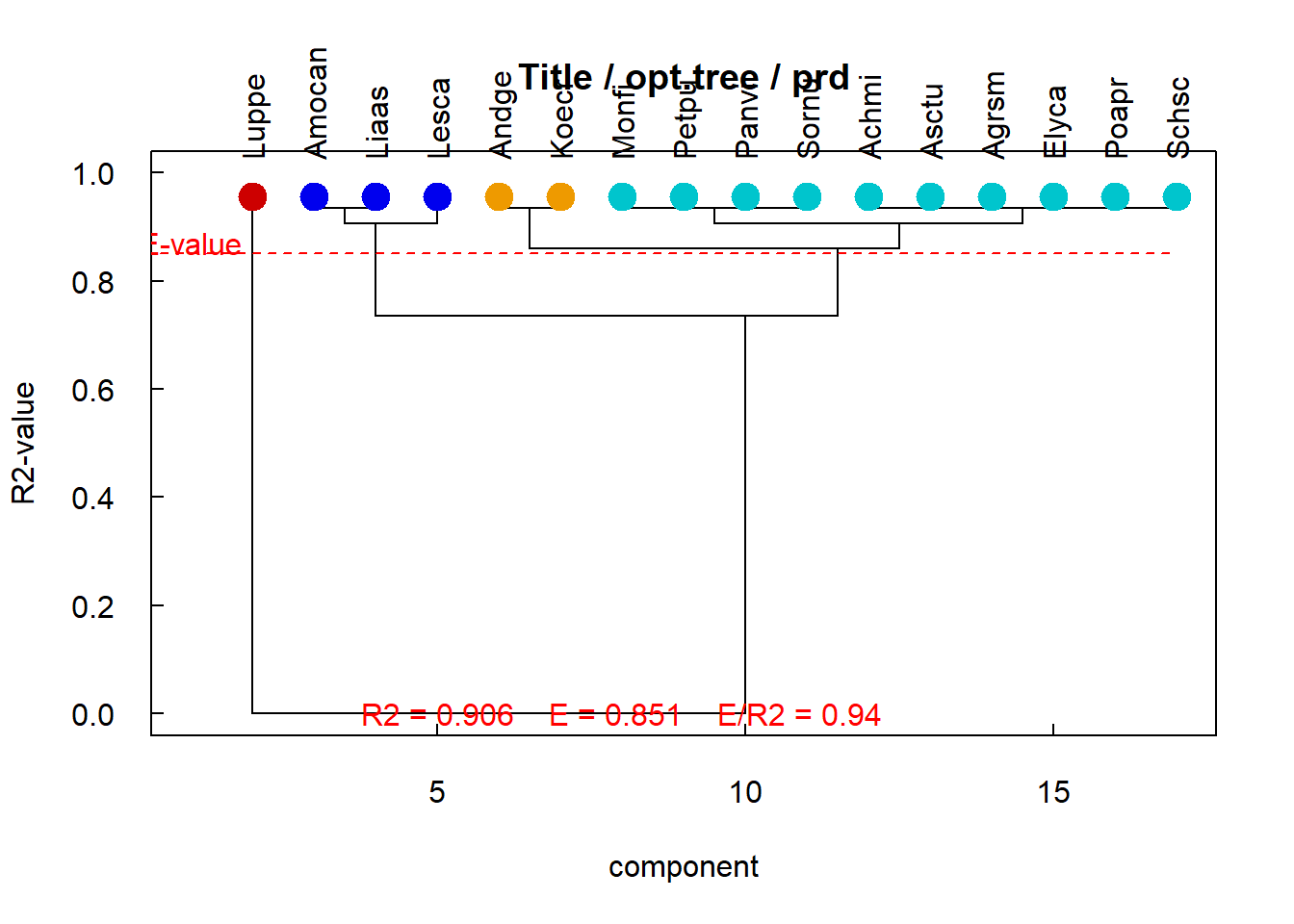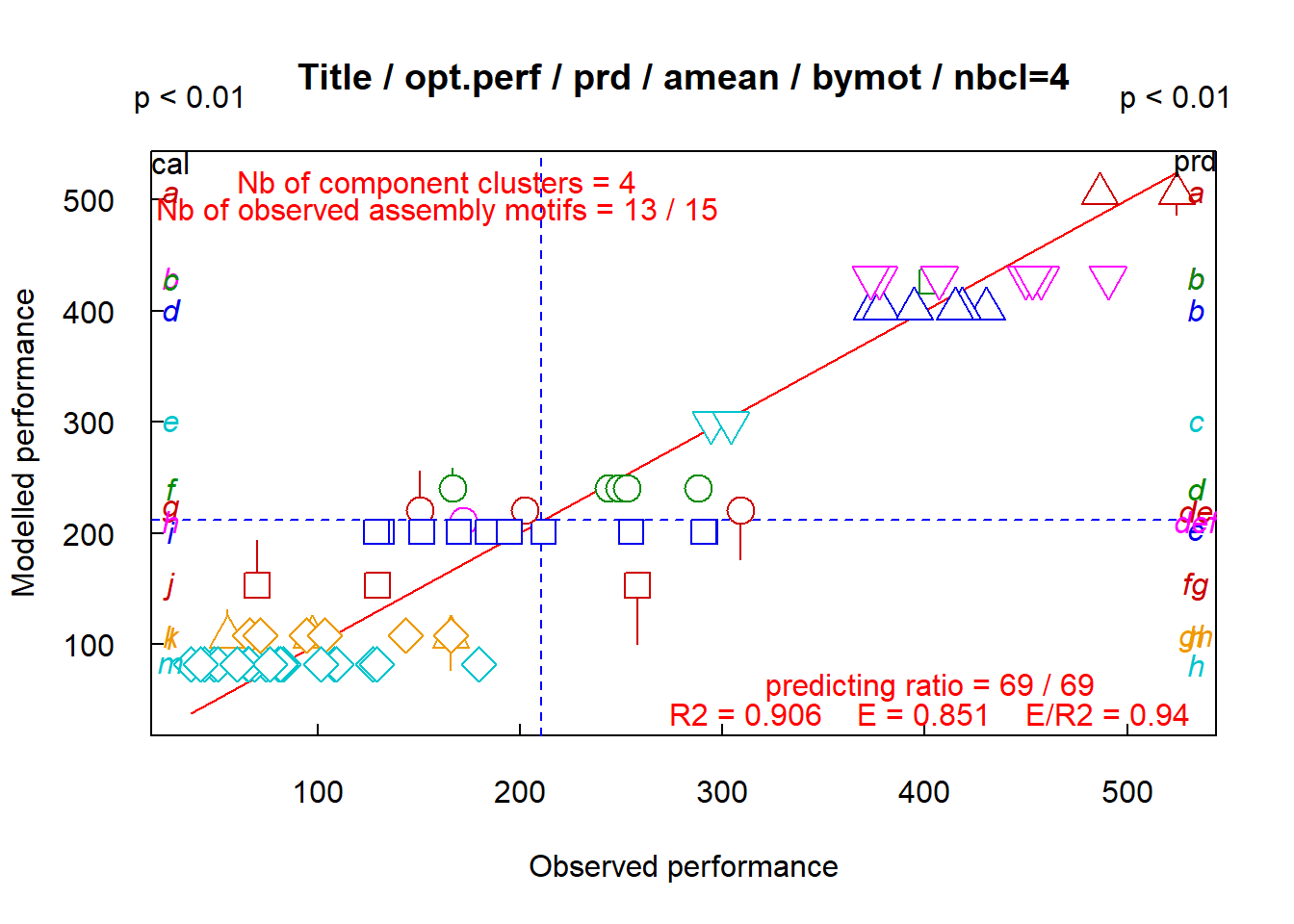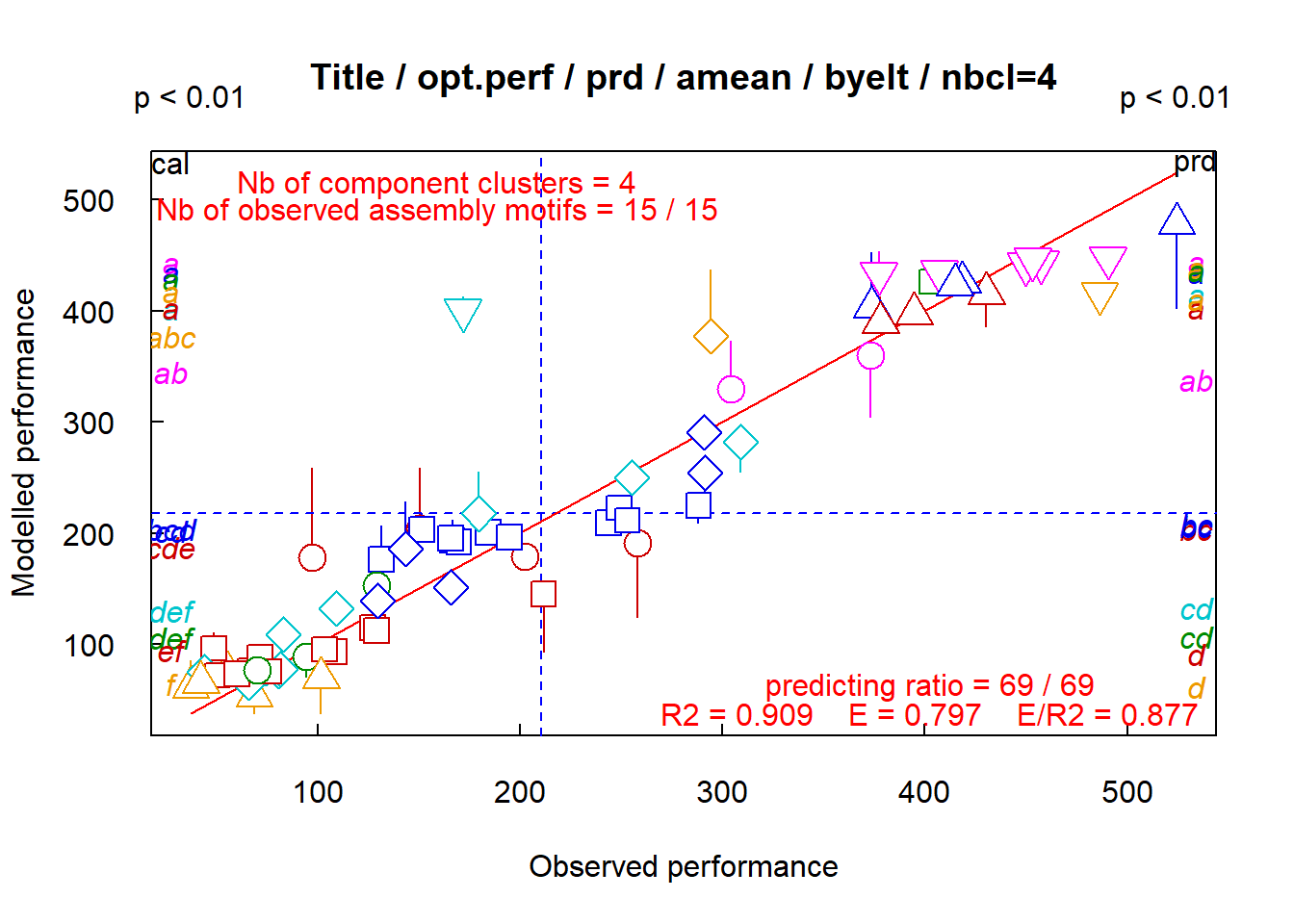Opt.model determines the model for predicting assemblage performance

Both the highest graphs correspond to opt.model = “bymot”, both the lowest graphs to opt.model = “byelt”. The resulting trees are different: the first redgroup contains Luppe in both the trees, the second blue-group contains Liaas and Lesca in both the trees, but differs by Amocan and Koecr, the third *gold“group contains Andge* in both the trees, but Koecr in bymot-tree and several other species in byelt-tree, etc…. The coefficients of determination are equivalent (R2 = 0.906 against 0.909), and the predictive ability of assemblage performances are more robust with opt.model = “bymot” than with opt.model = “byelt” (E = 0.851 against 0.797, then E/R2 = 0.940 against 0.877). However, our experiment suggests that opt.model = “byelt” gives the most likely result.

The option opt.mean

opt.mean determines the formula to use in averaging. The option can be “amean” or “gmean”. Functional clustering is based on computations of mean performances of assemblages, differently partitioned. The mean formula to use depends on the distribution of assemblage performance: it can shift a little the resuls.

• If opt.mean = “amean”, mean performances are computed using an arithmetic formula.

• If opt.mean = “gmean”, mean performances are computed using a geometric formula.

res <- fclust(dat.2004, nbElt, opt.mean = "amean")
fclust_plot(res, opt.tree = list("prd"), opt.perf = list("prd", "aov", pvalue = 0.01))

res <- fclust(dat.2004, nbElt, opt.mean = "gmean")
fclust_plot(res, opt.tree = list("prd"), opt.perf = list("prd", "aov", pvalue = 0.01))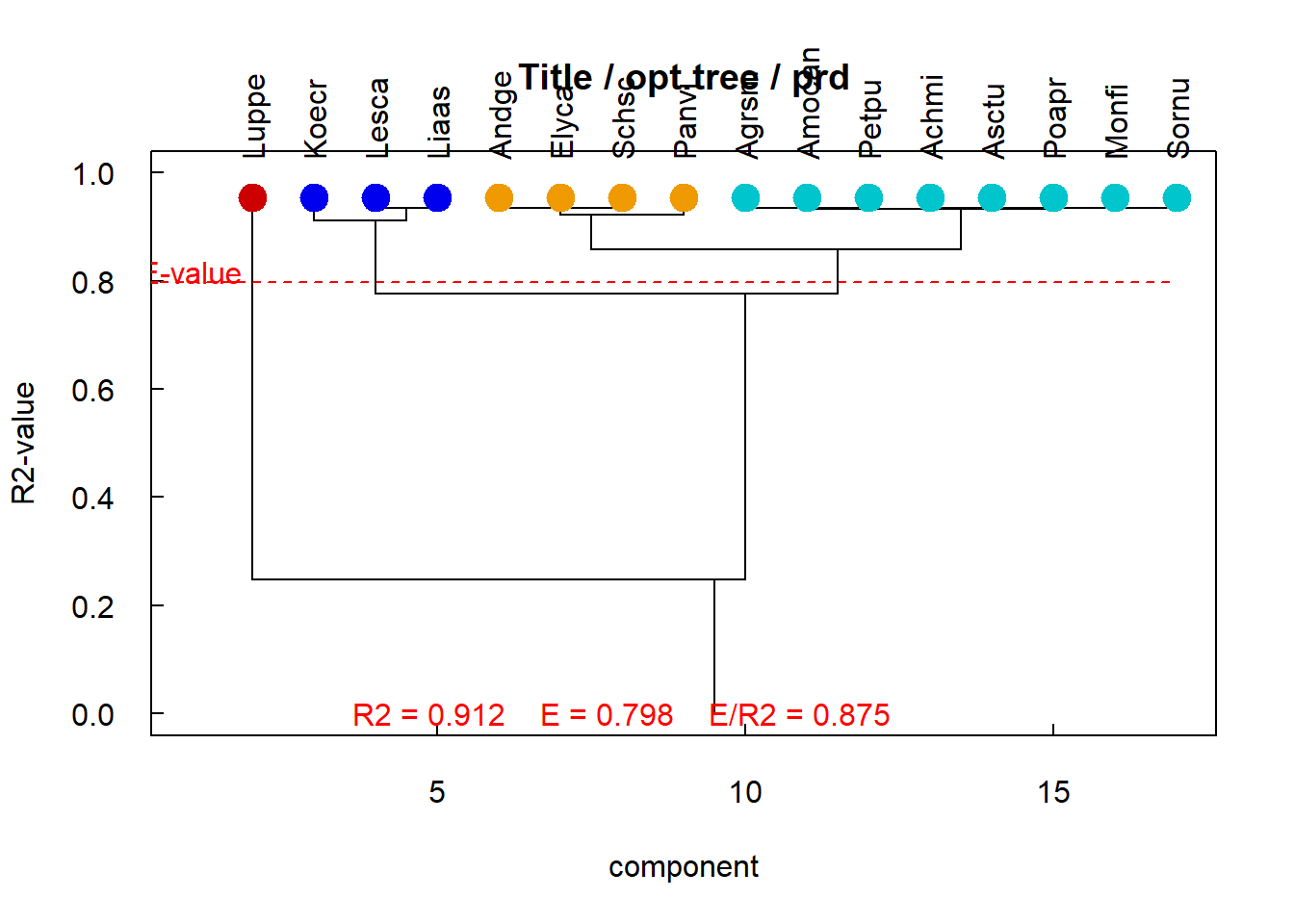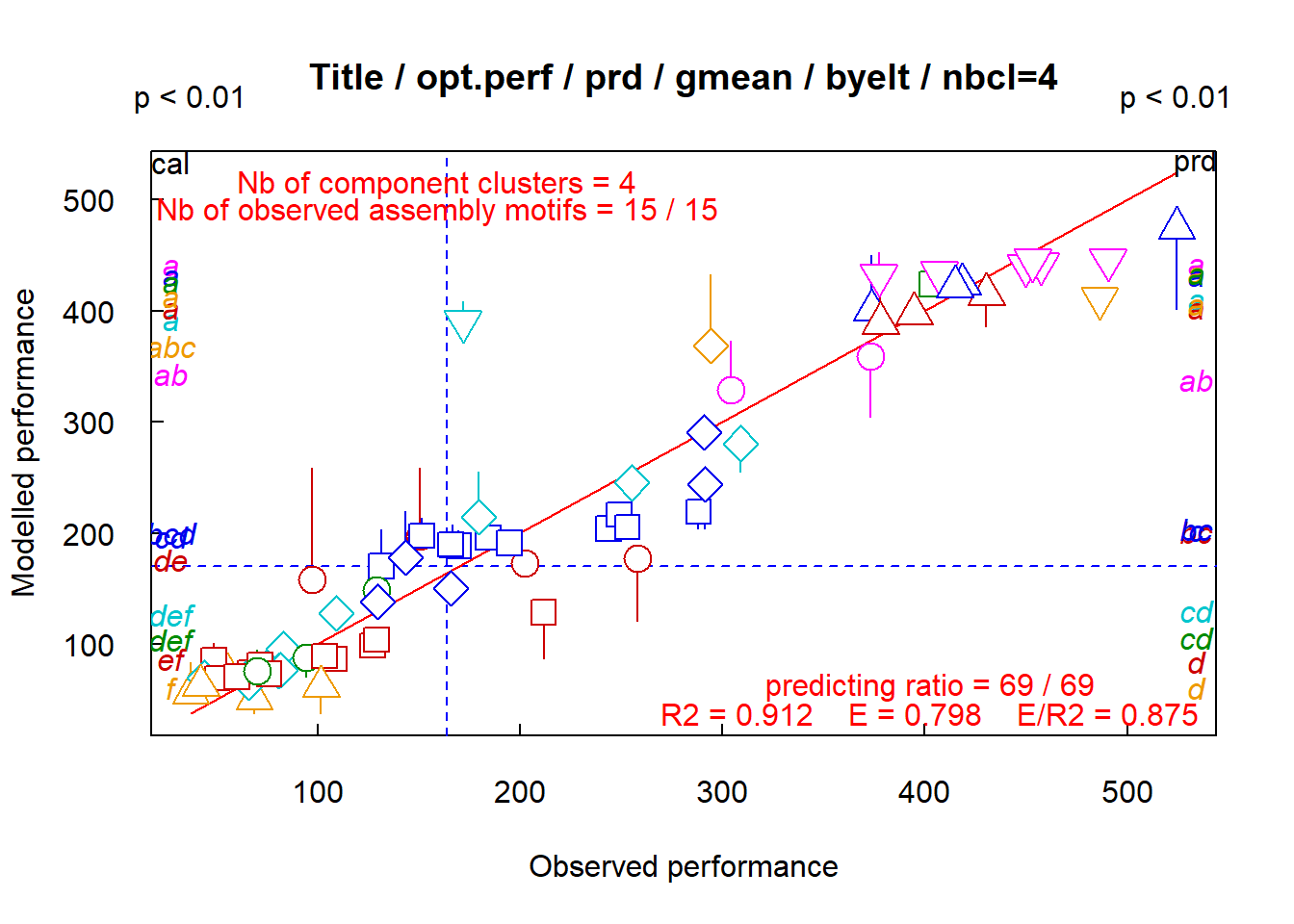Opt.mean determines the formula to use in averaging

The left graph corresponds to opt.mean = “amean”, the right graph to opt.mean = “gmean”. The resulting trees are the same, and the model goodness-of-fit (R2 = 0.909 against 0.940; E = 0.797 against 0.798) are not significantly different.

The option opt.jack

opt.jack determines the method of cross-validation. By default (opt.jack = FALSE), the performance of each assemblage is predicted by a Leave-One-Out method: the performance of each assemblage is predicted as the mean performance of assemblages that share a same assembly motif, except the only assemblage to predict. If the number of assemblages that share a same assembly motif is large, Leave-One-Out method is time-consuming. It is more convenient to switch towards a jackknife method (opt.jack = TRUE): the performances of assemblages that belong to each subset are predicted as the mean performance of assemblages of other subsets, except the assemblage subset to predict. jack then specifies how to divide the assemblage collection. jack is an integer vector of length 2: the first integer specifies the size of subset, the second integer specifies the number of subsets.

Note

Note that some computations are time-consuming. To facilitate the monitoring of the smooth running of the computations, informations are written on the Console and graphs are drawn on the Plots panel. The writting are activated or deactivated by the “verbose” option.

getOption("verbose")
#>  FALSE